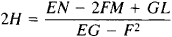# Mean Curvature

Also found in: Wikipedia.

## mean curvature

[¦mēn ′kər·və·chər]
(mathematics)
Half the sum of the principal curvatures at a point on a surface.

## Mean Curvature

The mean curvature of a surface at a given point P on the surface is equal to the average of the principal curvatures of the surface at the point (seeDIFFERENTIAL GEOMETRY). If E, F, and G are the coefficients of the first fundamental quadratic form of the surface and L, M, and N are the coefficients of the surface’s second fundamental quadratic form, then the mean curvature H can be computed from the formulaIf the mean curvature is equal to zero at every point of the surface, then the surface is known as a minimal surface.

Mentioned in ?
References in periodicals archive ?
In this study, mean curvature is employed to characterize the surfaces to be compared.
Assume that the mean curvature form is bounded and coclosed.
For readers who have completed their study of linear elliptic differential equations and intend to explore nonlinear ones, Han discusses quasilinear and fully nonlinear equations, focusing on two important nonlinear elliptic differentials closely related to geometry, the mean curvature equation and the Monge-Ampere equation.
Hopf, a powerful analytic tool which is often used in the theory of minimal or constant mean curvature hypersurfaces.
The remaining nine parameters are derived from the Mean Curvature View namely Max Mean curvature, Max Mean Curvature Xmm, Max Mean curvature Ymm, Centroid Xmm, Centroid Ymm, Convexity Ymm, Convexity, Mean I-S, Mean IN-ST, Mean IN-SN.
Our method to get the estimates parallels that of Escobar and Garcia () in the problem of prescribed mean curvature on the boundary of the ball.
do Carmo: Hypersurfaces with constant mean curvature in sphere, Proc.
The main extrinsic invariant is the squared mean curvature and the main intrinsic invariants include the classical curvature invariants, namely the scalar curvature and the Ricci curvature; and the well known modern curvature invariant, namely Chen invariant .
If the mean curvature H of the affine translation surface r(x, y) in [E.
One should keep in mind that the length of a fiber (with small cross-section) is approximately proportional to the integral of the mean curvature of the fiber.

Site: Follow: Share:
Open / Close